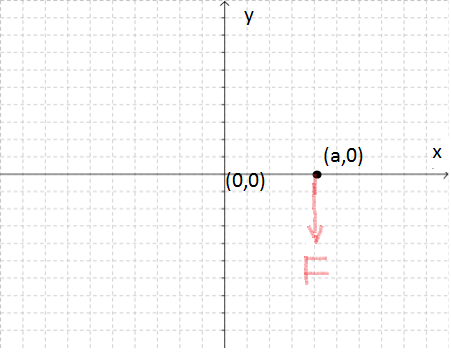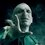# Physics Doubts: What is Rotation and whether torque is responsible or not?Doubt 1 : In the figure shown , a particle is initially placed at (a,0) and then the force F is applied in the direction of negative y axis. I am confused about what will be the torque acting on the particle about the y axis. It seems that it is 0 because the particle is not rotating about the y axis, but mathematically the cross product i x (-j) is not 0 (but the component of torque in the direction of y axis is indeed 0, and that makes me still more confused).

Doubt 2: What is the relation between torque acting on a body about an axis and the body rotating about that axis , i.e , can a body be in rotational motion about an axis only when torque acts on it (and moreover when the torque has non 0 component in the direction of axis), or not. [In Uniform Circular motion(which I think is rotational motion about the symmetric axis perpendicular to the plane) no such torque acts, so is it rotational motion or not]

Doubt 3 : What is the basic definition of AXIS OF ROTATION. Is the statement " axis of rotation is always perpendicular to the plane of rotation" correct?

Also, what is the best definition of Rotational Motion about an axis ?Note by Abhijeet Verma
5 years, 12 months ago

This discussion board is a place to discuss our Daily Challenges and the math and science related to those challenges. Explanations are more than just a solution — they should explain the steps and thinking strategies that you used to obtain the solution. Comments should further the discussion of math and science.

When posting on Brilliant:

• Use the emojis to react to an explanation, whether you're congratulating a job well done , or just really confused .
• Ask specific questions about the challenge or the steps in somebody's explanation. Well-posed questions can add a lot to the discussion, but posting "I don't understand!" doesn't help anyone.
• Try to contribute something new to the discussion, whether it is an extension, generalization or other idea related to the challenge.

MarkdownAppears as
*italics* or _italics_ italics
**bold** or __bold__ bold
- bulleted- list
• bulleted
• list
1. numbered2. list
1. numbered
2. list
Note: you must add a full line of space before and after lists for them to show up correctly
paragraph 1paragraph 2

paragraph 1

paragraph 2

[example link](https://brilliant.org)example link
> This is a quote
This is a quote
    # I indented these lines
# 4 spaces, and now they show
# up as a code block.

print "hello world"
# I indented these lines
# 4 spaces, and now they show
# up as a code block.

print "hello world"
MathAppears as
Remember to wrap math in $$ ... $$ or $ ... $ to ensure proper formatting.
2 \times 3 $2 \times 3$
2^{34} $2^{34}$
a_{i-1} $a_{i-1}$
\frac{2}{3} $\frac{2}{3}$
\sqrt{2} $\sqrt{2}$
\sum_{i=1}^3 $\sum_{i=1}^3$
\sin \theta $\sin \theta$
\boxed{123} $\boxed{123}$

Sort by:

Hi @Abhijeet Verma
You are basically stuck in the fundamentals of rotations which many teachers even avoid to discuss..!!
let me give you few points to ponder upon,...
1) Particles doesnot rotate!!! they can revolve but they can't rotate..!!
2) Torque about a point and torque about an axis are two different things..Means toque about origin and torque about Y axis are two different things..
3) In general torque is not exactly related with angular velocity..it is related with angular momentum..i.e. Net torque about a point equals rate of change of angular momentum

To define rotation you need to have a rigid body first, then draw a random line on the body, if with time during the motion this line rotates then the body is said to be in rotation.

To define axis of rotation:
Instantaneous axis of rotation is an axis about which all the particles on a rigid body can be considered to be moving in a circular path at that instant of time. This axis will carry the centers of those circular motions. If the rotation is pure then the axis of rotation is fixed in space other wise it may change from one place to another.

- 5 years, 11 months ago

If a point object is revolving around a fixed axis , won't it be rotational motion ?(I actually thought that revolving is in a way rotating)

- 5 years, 11 months ago

If we say that rigid body rotation about an axis is the motion in which all the points on the body move a same angle in a same interval of time, then pure revolution of a body about an axis should indeed be rotation. Also, keeping aside , particle body has only one point, so why isn't its revolution- rotation? @Rohit Gupta sir

- 5 years, 11 months ago

@Rohit Gupta sir, I am still awaiting a reply

- 5 years, 11 months ago

The way earth goes around sun is said to be revolution and the way it goes about its axis is said to be rotation.
The way rotation is defined we cannot have it for a particle. A body is said to be rotating if any line drawn on the body changes its orientation with time..

- 5 years, 11 months ago

u have done the cross product for the particle revolving about origin or we can that z axis will be its axis of rotation for doubt 2: for initiating a rotational motion u need to have torque but rotational motion doesnt implies torque because a body can rotate with uniform velocity(so no torque is acting) so, torque acting on a body about an axis = the body rotating about that axis with VARYING angular velocity torque NOT acting on a body about an axis = the body rotating about that axis with uniform angular velocity

- 5 years, 11 months ago

Doubt 2 : Torque increases angular mumentum or velocity. If net torque = 0, $\omega$ remains constant.

- 5 years, 10 months ago